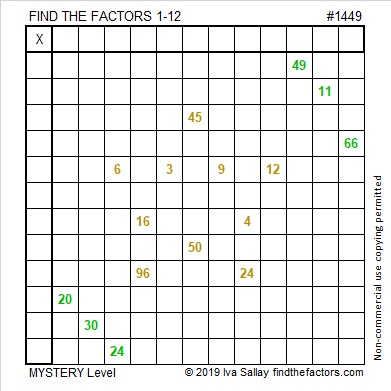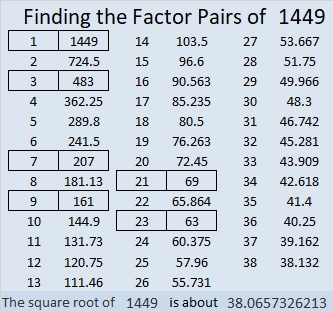# 1449 Christmas Star

If you’ve ever wished you knew the multiplication table better, then make that wish upon this Christmas star. If you use logic and don’t give up,  then you can watch your wish come true!I number the puzzles to distinguish them from one another. That star puzzle is way too big for a factor tree made with its puzzle number:Here’s more about the number 1449:

• Prime factorization: 1449 = 3 × 3 × 7 × 23, which can be written 1449 = 3² × 7 × 23
• 1449 has at least one exponent greater than 1 in its prime factorization so √1449 can be simplified. Taking the factor pair from the factor pair table below with the largest square number factor, we get √1449 = (√9)(√161) = 3√161
• The exponents in the prime factorization are 2, 1, and 1. Adding one to each exponent and multiplying we get (2 + 1)(1 + 1)(1 + 1) = 3 × 2 × 2 = 12. Therefore 1449 has exactly 12 factors.
• The factors of 1449 are outlined with their factor pair partners in the graphic below.1449 is the difference of two squares in 6 different ways:
725² – 724² = 1449
243² – 240² = 1449
107²-100² = 1449
85² – 76² = 1449
45² – 24² = 1449
43² – 20² = 1449

# Why is 1441 a Star?

1441 is the 16th star number. If you look at the tiny squares that make up each triangle in the star, you might notice that each of those triangles is made with 15 rows of squares. Thus each triangle represents the 15th triangular number.A six-pointed star is really just a 12-sided figure better known as a dodecagon.

Since I recently wrote that figurate numbers can be found using triangular numbers, I wondered if centered figurate numbers can make the same claim. Yes, they can!

Centered Figurate Numbers are also called Centered Polygonal Numbers and can be easily calculated from the triangular numbers.Here are some more facts about the number 1441:

• 1441 is a composite number.
• Prime factorization: 1441 = 11 × 131
• 1441 has no exponents greater than 1 in its prime factorization, so √1441 cannot be simplified.
• The exponents in the prime factorization are 1, and 1. Adding one to each exponent and multiplying we get (1 + 1)(1 + 1) = 2 × 2 = 4. Therefore 1441 has exactly 4 factors.
• The factors of 1441 are outlined with their factor pair partners in the graphic below.1441 is a palindrome with an even number of digits so it is divisible by 11.

1441 is also a palindrome in some other bases, namely, base 6, base 30, base 32, and base 36.

# 1093 is a STAR!

(12 × 13 × 14)/2 = 1092 and that makes 1093 a STAR!1092 had more than its fair share of factors forcing 1093 to have only two factors, but that’s okay because 1093 is a beautiful STAR! Why is it a STAR? Because 1093 is one more than 12 times the 13th triangular number. Do you see those 12 triangles in the image above? Each of them has the same number of tiny squares. The yellow square in the center is the plus one that completes the star.

There are some other reasons why 1093 deserves a gold star:

Not every prime number is in a twin prime, but 1091 and 1093 are twin primes. Even fewer are part of a prime triplet, but those twin primes are part of TWO prime triplets: the 31st and the 32nd! That’s because 1087, 1091, 1093, and 1097 are all prime numbers.

• 1093 is a prime number.
• Prime factorization: 1093 is prime.
• The exponent of prime number 1093 is 1. Adding 1 to that exponent we get (1 + 1) = 2. Therefore 1093 has exactly 2 factors.
• Factors of 1093: 1, 1093
• Factor pairs: 1093 = 1 × 1093
• 1093 has no square factors that allow its square root to be simplified. √1093 ≈ 33.03329

How do we know that 1093 is a prime number? If 1093 were not a prime number, then it would be divisible by at least one prime number less than or equal to √1093 ≈ 33.1. Since 1093 cannot be divided evenly by 2, 3, 5, 7, 11, 13, 17, 19, 23, 29 or 31, we know that 1093 is a prime number.1093 is the sum of two squares:
33² + 2² = 1093

It is the hypotenuse of a primitive Pythagorean triple:
132-1085-1093 calculated from 2(33)(2), 33² – 2², 33² + 2²

Here’s another way we know that 1093 is a prime number: Since its last two digits divided by 4 leave a remainder of 1, and 33² + 2² = 1093 with 33 and 2 having no common prime factors, 1093 will be prime unless it is divisible by a prime number Pythagorean triple hypotenuse less than or equal to √1093 ≈ 33.1. Since 1093 is not divisible by 5, 13, 17, or 29, we know that 1093 is a prime number.

3⁶ + 3⁵ + 3⁴ + 3³ + 3² + 3¹ + 3⁰ = 1093 so 1093 is represented by 1111111 in BASE 3. That also means that 2(1093) + 1 = 3⁷.

1093 is a palindrome in two bases:
1G1 in BASE 26 (G is 16 base 10) because 26² + 16(26) + 1 = 1093, and
1B1 in BASE 28 (B is 11 base 10) because 28² + 11(28) + 1 = 1093

From Stetson.edu and Wikipedia, we learn something quite unique about 1093 – that it is the smaller of the two known Wieferich primes, 1093 and 3511.

Wow! 1093 truly is a STAR!

# 987 Christmas Star

Today’s puzzle is a lovely Christmas star whose golden beams shine throughout the dark night. Solving this puzzle could also enlighten your mind.Print the puzzles or type the solution in this excel file: 10-factors-986-992

987 is made from three consecutive numbers so it is divisible by 3.

It is also the sixteen number in the Fibonacci sequence:
1, 1, 2, 3, 5, 8, 13, 21, 34, 55, 89, 144, 233, 377, 610, 987, . . .

987 is a palindrome when written in base 11 or base 29:
818 in BASE 11 because 8(121) + 1(11) + 8(1) = 987
151 in BASE 29 because 1(29²) + 5(29) + 1(1) = 987

• 987 is a composite number.
• Prime factorization: 987 = 3 × 7 × 47
• The exponents in the prime factorization are 1, 1, and 1. Adding one to each and multiplying we get (1 + 1)(1 + 1)(1 + 1) = 2 × 2 × 2 = 8. Therefore 987 has exactly 8 factors.
• Factors of 987: 1, 3, 7, 21, 47, 141, 329, 987
• Factor pairs: 987 = 1 × 987, 3 × 329, 7 × 141, or 21 × 47
• 987 has no square factors that allow its square root to be simplified. √987 ≈ 31.416556# 332 and a Christmas Star

• 332 is a composite number.
• Prime factorization: 332 = 2 x 2 x 83 which can be written 2² x 83
• The exponents in the prime factorization are 1 and 1. Adding one to each and multiplying we get (2 + 1)(1 + 1) = 3 x 2 = 6. Therefore 332 has exactly 6 factors.
• Factors of 332: 1, 2, 4, 83, 166, 332
• Factor pairs: 332 = 1 x 332, 2 x 166, or 4 x 83
• Taking the factor pair with the largest square number factor, we get √332 = (√4)(√83) = 2√83 ≈ 18.221Merry Christmas! This is a rather easy level 5 puzzle so I’m sharing it instead of a level 4 puzzle today.Print the puzzles or type the factors on this excel file:  10 Factors 2014-12-22

The Night Wind excitedly asked a little lamb and the whole world, “Do you see what I see?” when it saw “a star, a star, dancing in the night” after the Christ child was born. Click here to read the complete lyrics of “Do You Hear What I Hear.”